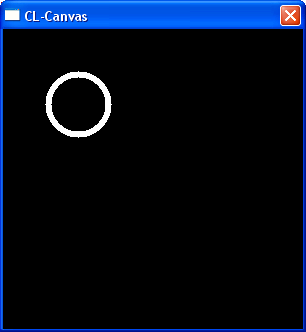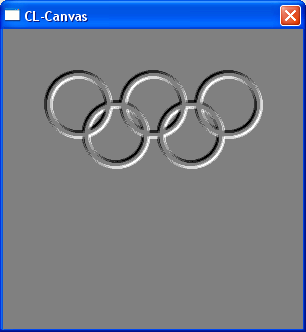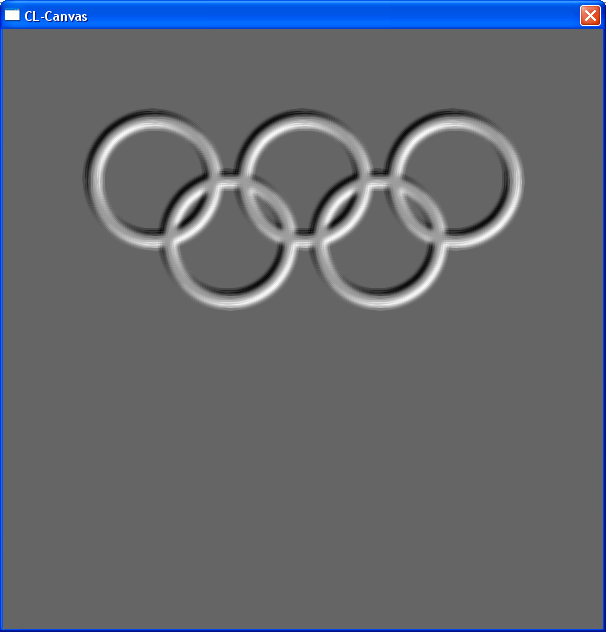# Functional Textures

Like with line drawings in Functional Geometry, you can generate nice textures when using functions, which creates functions, which takes the current point for an image to calculate as a parameter. For interactive testing I'm using CL-Canvas.

```(clc:show-canvas 300 300)
(defparameter c1 (circle :x0 -0.5 :y0 -0.5 :radius 0.2 :line-width 0.02))
(paint c1)
``````(defparameter c2 (circle :x0  0.0 :y0 -0.5 :radius 0.2 :line-width 0.02))
(defparameter c3 (circle :x0  0.5 :y0 -0.5 :radius 0.2 :line-width 0.02))
(defparameter c4 (circle :x0  -0.25 :y0 -0.3 :radius 0.2 :line-width 0.02))
(defparameter c5 (circle :x0  0.25 :y0 -0.3 :radius 0.2 :line-width 0.02))
(defparameter olympic (add c1 c2 c3 c4 c5))
(paint olympic)
```Convert to black and white:

```(defparameter olympic-bw (black-white :function olympic :limit 0.5))
(paint olympic-bw)
``````(defparameter embossed-rings (emboss :function olympic-bw :sample-radius 0.01))
(paint embossed-rings)
```Fill the center again with the original circle, to make it brighter:

```(defparameter added-rings (add olympic-bw embossed-rings))
```And finally some blur for a more naturally looking:

```(defparameter olympic-rings (blur :function added-rings :sample-radius 0.007))
(paint olympic-rings)
```Because it is calculated by formulas, you can scale it lossless to every size:And here are the definition of paint and the other functions:

```(defun circle (&key x0 y0 radius line-width)
(lambda (x y)
(let ((xc (- x x0))
(yc (- y y0)))
(let ((r2 (sqrt (+ (* xc xc) (* yc yc)))))
(if (and (>= r2 (- radius line-width))
1.0
0.0)))))

(defun convolution (&key function matrix sample-radius divisor)
(lambda (x y)
(let ((sum 0.0))
(loop for xo from -1 to 1 do
(loop for yo from -1 to 1 do
(incf sum (* (aref matrix (1+ yo) (1+ xo))
(funcall function
(setf sum (/ sum divisor))
sum)))

(convolution :function function
:matrix '#2a((0.7 1.0 0.7)
(1.0 1.0 1.0)
(0.7 1.0 0.7))
:divisor 9.0))

(convolution :function function
:matrix '#2a(( 1.0  0.7  0.0)
( 0.7  0.0 -0.7)
( 0.0 -1.0 -0.7))
:divisor 1.0))

(lambda (x y)
(let ((sum 0))
(loop for function in functions do
(incf sum (funcall function x y)))
sum)))

(defun black-white (&key function limit)
(lambda (x y)
(if (> (funcall function x y) limit)
1.0
0.0)))

(defun paint (function)
(let ((data (make-array (list clc:*height* clc:*width*)))
(min-date 1.0e10)
(max-date -1.0e10))
(loop for y from 0 below clc:*height* do
(loop for x from 0 below clc:*width* do
(let ((date (funcall function
(- (* (/ x clc:*width*) 2) 1.0)
(- (* (/ y clc:*height*) 2) 1.0))))
(setf (aref data y x) date)
(when (< date min-date) (setf min-date date))
(when (> date max-date) (setf max-date date)))))
(loop for y from 0 below clc:*height* do
(loop for x from 0 below clc:*width* do
(let ((normalized-date (/ (- (aref data y x) min-date)
(- max-date min-date))))
(let ((c (round (* 256 normalized-date))))
(when (> c 255) (setf c 255))
(when (< c 0) (setf c 0))
(setf (clc:framebuffer-point x y)
(logior c (ash c 8) (ash c 16)))))))
(clc:repaint)))
```

27. March 2005, Frank Buß